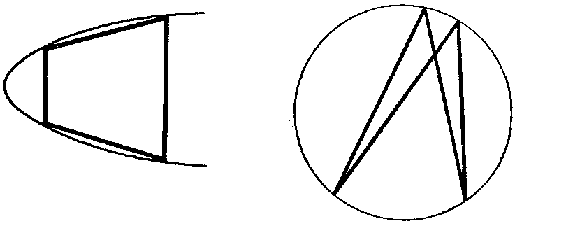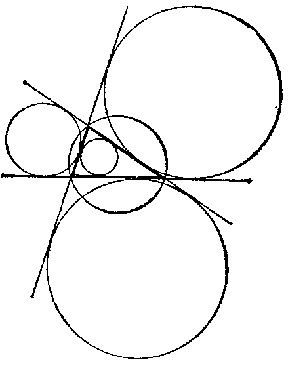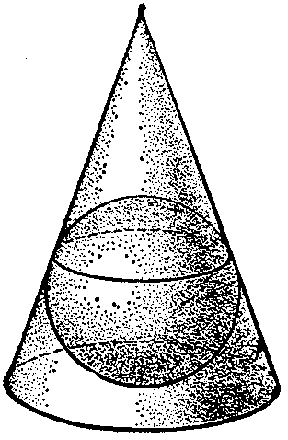# Inscribed and circumscribed figures

A polygon is said to be inscribed in a convex curve, while the curve is said to circumscribe the polygon, if all vertices of the polygon lie on the curve (Fig. a).Figure: i051250a

A polygon is said to be circumscribed about a convex curve, while the curve is said to inscribe (or externally inscribe, escribe) the polygon, if all sides of the polygon (extended if necessary) are tangent to the curve. The curve most frequently considered in this connection is a circle. For instance, any triangle has one circumscribed circle and four inscribed circles, three of which are externally inscribed (Fig. b).Figure: i051250bFigure: i051250c

Inscribed and circumscribed figures are also considered in space. In such a case the polygon is replaced by a polyhedron, while the convex curve is replaced by a convex surface, usually by a sphere. One may also speak of a cone inscribed in a sphere, a sphere inscribed in a cone, etc. (Fig. c).

How to Cite This Entry:
Inscribed and circumscribed figures. Encyclopedia of Mathematics. URL: http://encyclopediaofmath.org/index.php?title=Inscribed_and_circumscribed_figures&oldid=53942
This article was adapted from an original article by A.B. Ivanov (originator), which appeared in Encyclopedia of Mathematics - ISBN 1402006098. See original article